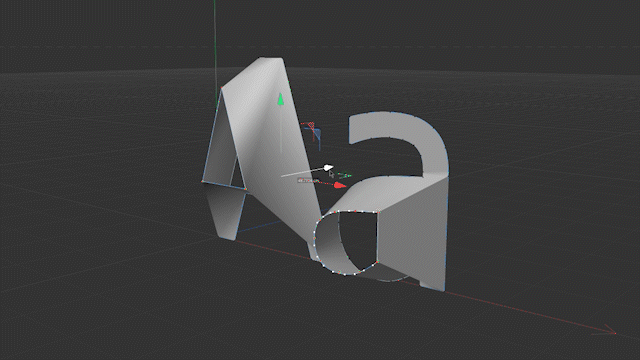# Condition that generator treats inner spline segment as a hole

Hi,

Out of curiosity, I would like to know under what conditions the inner segment of a spline behaves as a hole.
If possible, I would like to reproduce this behavior in Python Generator.
Any hints would be appreciated.Hello @kng_ito,

Thank you for reaching out to us. In general, we do not expose the implementations details of Cinema 4D and curiosity alone is unfortunately not a sufficient reason to make an exception.

With that being said, and without having had a look at how the polygon object generators like the extrude object handle this, here is how you could determine for the spline segments S and T if T is a hole of S or not.

1. Calculate the mean point p and mean normal n for each set S and T. In the operation_flatten_polygons_s26.py example I showed how to calculate the mean plane for a set of polygons, doing it for an ordered list of line segments will almost be the same. You just must derive orientation, a.k.a., the normals, from the vertex order of the spline segment here.
2. Define a tolerance d upon which (p_T, n_T) is not a hole of (p_S, n_S) anymore.
• A simple way to do it would be to test the angle between both normals for not being larger than d_orientation, e.g., 30°, and the distance between both points for not being larger than d_distance, e.g., 100 units.
• You could define d_distance by computing the 2D bounding box of the vertices of S projected into their mean plane. You could then set d_distance to be equal to the smallest component of the bounding box. I.e., a hole is not a hole anymore whenT farther away from S than S is tall or wide in its mean plane projection.

Cheers,
Ferdinand

MAXON SDK Specialist
developers.maxon.net

Hi @ferdinand,

So the normal direction of the generated polygons was the point!
Thank you for answering my curiosity and explaining how to reproduce the behavior.

Hey @kng_ito,

So the normal direction of the generated polygons was the point!

It depends a bit on what you mean by normal direction, but generally speaking, normals only play a minor role here.

Wenn all segments would always lie nicely inside a plane, it would be easy. Just compute the mean point for each segment and then come up with some cockamamie rule when you would consider two segments not being in a shell-hole relation (as I did above with 'a hole is not a hole anymore when T farther away from S than S is tall or wide').

In practice things do not always lie nicely in a plane. So, you then must first project them there, find their mean plane. So, the 'point' would be finding the mean plane / dimensionality reduction. I cooked up an small example below.

Cheers,
Ferdinand

Result (the plane gizmos are a bit buggy and sliding, did not debug this. But that is why I turn them off):The file:
spline_hole.c4d

MAXON SDK Specialist
developers.maxon.net